﻿ Analytical Comparison of Swarm Intelligence Optimization versus Behavioral Learning Concepts Adopted by Neural Networks (An Overview)

### Analytical Comparison of Swarm Intelligence Optimization versus Behavioral Learning Concepts Adopted...

Hassan M. H. Mustafa

## Analytical Comparison of Swarm Intelligence Optimization versus Behavioral Learning Concepts Adopted by Neural Networks (An Overview)

Hassan M. H. MustafaCurrently with Al-Baha University, Faculty of Eng., Computer Eng. Department (K.S.A), On leave from Faculty of Specified Education-Banha University Egypt

### Abstract

Generally, in nature, non-human creatures perform adaptive behaviors to external environment they are living in. i.e. animals have to keep alive by improving their intelligent behavioral ability to be adaptable to their living environmental conditions. This paper presents an investigational comparative overview on adaptive behaviors associated with two diverse biological systems (Neural and Non-Neural). In more details, intelligent behavioral performance of Ant Colony System () in order to reach optimal solution of Traveling Sales-man Problem (TSP) is considered. That's investigated herein versus concepts of adaptive behavioral learning concerned with some animals (cats, dogs, and rats), in order to keep survive. More precisely, investigations of behavioral observations tightly related to suggested animals, supposed to obey discipline of biological information processing. So, Artificial Neural Network () modeling is a relevant tool to investigate such biological system observations. Moreover, an illustrative brief of optimal intelligent behaviors to solve is presented. Additionally, considering effect of noisy environment on learning convergence, an interesting analogy between both proposed biological systems is introduced. Finally, performance of three learning algorithms shown to be analogously in agreement with behavioral concepts of both suggested biological systems' performance.

• Hassan M. H. Mustafa. Analytical Comparison of Swarm Intelligence Optimization versus Behavioral Learning Concepts Adopted by Neural Networks (An Overview). American Journal of Educational Research. Vol. 3, No. 7, 2015, pp 800-806. http://pubs.sciepub.com/education/3/7/2
• Mustafa, Hassan M. H.. "Analytical Comparison of Swarm Intelligence Optimization versus Behavioral Learning Concepts Adopted by Neural Networks (An Overview)." American Journal of Educational Research 3.7 (2015): 800-806.
• Mustafa, H. M. H. (2015). Analytical Comparison of Swarm Intelligence Optimization versus Behavioral Learning Concepts Adopted by Neural Networks (An Overview). American Journal of Educational Research, 3(7), 800-806.
• Mustafa, Hassan M. H.. "Analytical Comparison of Swarm Intelligence Optimization versus Behavioral Learning Concepts Adopted by Neural Networks (An Overview)." American Journal of Educational Research 3, no. 7 (2015): 800-806.

 Import into BibTeX Import into EndNote Import into RefMan Import into RefWorks

1234
Prev Next

### 1. Introduction

Recently, research work for investigational analysis of behavioral intelligence & learning phenomena, is considered as an interdisciplinary challenging issue.. Such research field is concerned with behavioral learning performance observed by two diverse non-human creatures (Neural and Non-Neural biological systems) . in this work The performance of non-neural system considered is the intelligent behavioral Ant Colony System () in order to reach optimal solution of Traveling Sales-man Problem (TSP) [2, 3, 4, 5]. Principles of biological information processing concerned with learning convergence for both bio-systems have been compared at [6, 7, 8]. Herein, this work presents an interesting comparative overview introduced at the field of swarm intelligence optimization , versus conceptual view concerned with other animal's behavioral learning phenomenon [10, 11, 12, 13]. In other words, an investigational view is presented to get insight with behavioral intelligence & animal learning phenomena, [1, 4, 5, 11]. In more details, both biological systems are respectively observed by: Ant Colony System ACS while solving Traveling Sales-man Problem (TSP)  and some other animal creatures interacting with environment .Therein at , these suggested non-human creatures are: cats, dogs, and rats. However, more recently, one selected rat's behavioral learning model has been introduced in comparison with ACS's behavioral learning model . Interestingly, as well as other animals is commonly behaved interactively with their external environment on the bases of biological hypothesis: "Creatures have a tendency to behave adaptively in order to keep survive." .

Briefly, analysis of obtained results by such recent research work leads to discovery of some interesting analogous relations among presented behavioral learning paradigms . That concerned with observed resulting errors, time responses, and noisy disturbed outputs, versus number of trials, training dataset vectors, and number of processing agents [14, 15]. As examples of such agents are: generations in evolutionary genetic algorithms [16, 17], neural cells in hippocampus brain area [18, 19, 20, 21], and ants in ACS. Any of proposed learning systems (modeled or natural) are differently classified as either neural or non neural bio-system, . That is in addition to different nature of measuring learning parameters directing systems to required optimal output .

However, it seems to observe diversity of behavioral learning curves performance (till reaching optimum state) for proposed biological systems, both are similar to each other (considering normalization of performance curves) [3, 12]. In other words, behavioral intelligence & learning performance phenomena carried out by both biological systems are characterized by there adaptive behavioral responses to their living environmental conditions. . So, those phenomena consider input stimulating actions provided by external environmental conditions versus adaptive reactions carried by creatures, [9, 10, 11, 13].

The rest of paper is organized as follows. At next section, revising of generalized ANN learning model for unsupervised learning paradigm is presented . A review for performance of some animals' adaptive behavioral phenomenon is shown at section three. At fourth section, detailed comparison between environmental noise effects on behavioral learning performance for both biological systems is illustrated. This effect is compared considering optical character recognition (OCR) performed by an autonomous ANN model versus ACS optimization for solving Traveling Sales-man Problem TSP. At fifth section, brief mathematical description for rat's reconstruction (pattern recognition) problem compared with ACS optimization process is introduced at . Obtained results by experimental animal learning work, genetic engineering algorithm, and a review of Karhunen-Loeve theory searching for principal component analysis (PCA) are presented at sixth section. By more details, three learning algorithms are considered, namely parallel genetic algorithm (for pattern classification) [16, 17], least mean square (LMS) algorithm of error correction, , and modified Hebbian algorithm (Oja's rule) applied to search for (PCA), [24, 25, 26]. All of these learning algorithms have shown to be performed analogously as ACS solving optimally TSP. Finally, some conclusions and valuable discussion are given at the last seventh section.

### 2. Revising of Ann Learning Model

The shown model at Figure 1 in below simulates observed behavioral learning phenomenon associated with non-human creatures. There observed learning performance is evaluated while there adaptive interaction with external environment they are living in. This figure originated on the basis of the work of Fukaya, M., et al. published in 1988 at .Download asVeiw figureFigures index
Figure 1. Block diagram of generalized ANN modeling for supervised and unsupervised paradigms, adapted from 

The error vector at any time instant (n) observed during learning processes (in case of supervised paradigm) is given by: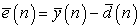(1)

Where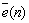: Error correcting signal controlling adaptively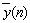: The output signal of the model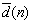: Numeric value(s) of the desired /objective parameter of learning process (generally as a vector).

Noting thatvector will not be taken into consideration for the case of unsupervised learning paradigm.

Referring to above Figure 1, following four equations describes dynamics of learning performance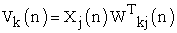(2)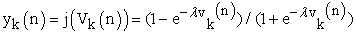(3)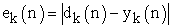(4)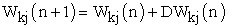(5)

Where:

X…… ………the input vector,

W……………the weight vector,

φ……………. the activation function,

y …………… the output,

ek ……………the error value,

λ …………… the gain factor suggested for ANN modeling, and

dk ……… …. .the desired output value.

Noting that ΔWkj(n) represents dynamical change of weight vector value.

The above four equations (2-5), are commonly applied for both (supervised, and unsupervised) learning paradigms. However, for our considerations to autonomously unsupervised learning paradigm ; synaptic connectivity changes at any time instant (n) , are given by synaptic weight vector value W , which dynamically presented as follows: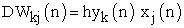(6)

where η is the learning rate value during unsupervised learning process. This equation (6), presents Hebbian unsupervised learning rule that relevant to simulate realistically behavioral animal learning.

### 3. Animals' Behavioral Learning Models

This section presents a review of behavioral learning phenomenon observed after a set of psycho-experimental work carried on two animal types: cats, and dogs. Those experiments had been performed - about one century ago- by Thorndike and Pavlov [9, 10] on cats, and dogs respectively. While analyzing of experimental results obtained , our presented study herein, came to conclusion that: all considered animals (cats, dogs, and rats) obey learning behaviorism approach based upon unsupervised learning paradigm .

Simulation of two experimental work obtained by Pavlov and Thorndike have been recently published [2, 3]. The following figure presence the normalized performance for both experimental works. This set of psycho-experimental work

referred to behavioral learning approach is adopted through trials and errors repeated cyclic steps (learning epochs),  Referring to original obtained results after the work of Thorndike & Pavlov, both results are normalized to unity value, resulting in two learning curves given at Figure 2.

Based on normalized experimental results, relationships between learning achievements (outputs) and subsequent training cycles are shown at Figure 2. Learning performance curves could be generally presented mathematically as a set of hyperbolic curves relation as follows: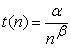(7)

Where α and β are arbitrary positive constants. These constants possibly have changeable values, in accordance with various individual differences performance.Download asVeiw figureFigures index
Figure 2. Illustrates the comparison between Pavlovian and Thorndikian work. The comparison considers normalized results after application of ANN simulation adapted from 

Similarly, the following figure given in below (Figure 3) illustrates learning performance of a rat while solving some reconstruction (pattern recognition) problem inside figure eight (8) maze. The graph shown at that figure represents recognition error versus number of place field cells (at hippocampus rat's brain area). That performance curve converged to fixed Cramer Rao. bound. (limiting value). However, this bounding limit (of minimum error value) is analogous to minimum time response corresponding to maximum number of trials limit by referring to above Figure 2. More precisely, by increasing of training cycles, shown learning algorithms tend to fixed limiting values (for time response). That is considering normalized value of number of trials and corresponding response times (for both experimental work of Pavlov and Thorndike). Conclusively, increasing of neuron cells cooperating in solving reconstruction (pattern recognition) problem, is analogous to increase in number of training cycles. However, as mean error in solution decreased (till reaching Cramer-Rao bounding limit), is analogous to minimum time response at Figure 2.Download asVeiw figureFigures index
Figure 3. The dashed line indicate the approach to Cramer-Rao bound based on Fisher information adapted from 

### 4. Noisy Environment Effect on Learning Performance

In natural real world, ideal (noiseless) learning environment is not realistically available. Usually, environmental observed learning and /or recognition data are vulnerable to be contaminated by either external or internal noisy conditions. Creatures having a tendency to behave adaptively in order to keep survive, they should be able to perform well recognition of all there environmental features of objects and/or other creatures (i.e. predators or victims….etc.). At this section, optical character recognition (OCR) process considered to simulate environmental learning and /or recognition performed by animals. Consequently, analysis of environmental noise illustrates its effects on both biological systems (during their adaptive behavioral learning) are comparatively introduced. Firstly, concerned with (OCR) process artificial neural network (ANN) applied for using self-organized learning paradigm. Secondly, deals Ant Colony Systems (ACS) used for solving traveling salesman problem (TSP) optimally.

4.1. Effect of Noise on OCR Processes 

In nature, optical character recognition OCR as well as pattern recognition processes observed to be carried out under no ideal environmental condition (under effect of noisy data). Interestingly, obtained simulation results for OCR.

Under different environmental noisy levels are given in a tabulated form at Table 1. Noting that, noise effect is measured by signal to noise ratio value (S/N) versus the number of training cycles (T) till reaching learning convergence. Conclusively, relation between number of training cycles' values and noisy levels of environmental data (for the case of unsupervised learning paradigm) is illustrated well at Figure 4.

Referring to that figure, learning convergence time T in cycles (n), inversely proportional to signal to noise ratio values (S/N).

#### Table 1. Illustrates the effect of noise on learning of convergence given by the relation between number of training cycles versus (s/n) ratioDownload asVeiw tableTables indexDownload asVeiw figureFigures index
Figure 4. Graphical presentation for learning performance under noisy conditions with reference to Table 1 adapted from 

Referring to Figure 5 given in below, some simulation results relating the number of training cycles (on the average), after learning convergence, versus learning rate values are shown.Download asVeiw figureFigures index
Figure 5. Illustrates the average (of statistical distribution) for learning response time (number of iteration cycles) for different learning rate values (eta)

It is worthy noting that statistical variations (on the average) relating learning rate values versus corresponding learning convergence (response) time. That time is measured by the number of iteration cycles. Referring to above Figure 5, obtained output results (of response time) corresponding to the learning rate values (0.1, 0.2, 0.4, 0.6, and 0.8), are given respectively, as (330, 170, 120, 80, and 40) iteration training cycles. Conclusively, convergence time (number of training cycles) is inversely proportional to the corresponding learning rate values. Moreover, relating both figures in the above (Figure 4 & Figure 5), it interesting to remark that under more noisy environmental conditions, learning rate tends to have lower value. Conversely, creatures that perform learning rate improvement by interaction with environment imply increase of their stored experience. Consequently, such creatures have become capable of responding spontaneously to input environmental stimuli in optimal manner, .

4.2. Effect of Noise on ACS Optimization

Referring to , the following noisy transition probability formula is shown at equation (8). However, details about more of its parametric variables are referred to above reference. This probability indicates the effect of noise power on the convergence of ant colony system to optimum solution.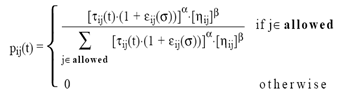(8)

Where β is a parameter determent the relative importance of pheromone substance versus distance (β > 0).

Allowed = {j: j ε tabuk} and εij (σ) is a noise function (random variable with zero mean and standard deviation σ).

α a pheromone decay parameter and ηij heuristic function.

This probability indicates the effect of noise power on the convergence of ant colony system to optimum solution. The performance of ACS to reach optimum solution under noisy condition is given in two forms. That are tabulated at Table 2, and graphically shown at Figure 6, as follows.

#### Table 2. Relation between average no of cycles needed to reach optimum solution versus signal to noise ratio valuesDownload asVeiw tableTables indexDownload asVeiw figureFigures index
Figure 6. Illustrates graphical presentation for reach optimal solution of TSP under noisy conditions by referring to Table 2

It is noticed that lower (S/N) values lead to significant worsening of the ACS performance to search optimum solution . The given above two Table 1 & Table 2 are comparatively illustrated graphically at Figure 3, and Figure 4 respectively. As a direct conclusion from above results is that interesting relation between cooperation and optimum solution convergence as follows. Referring to Figure 7 shown in below, the relation between tour lengths versus the CPU time is given. It is observed the effect of ant cooperation level on reaching optimum (minimum tour). Obviously, as level of cooperation among ants increases (better communication among ants) the CPU time needed to reach optimum solution is decreased. So, that optimum solution is observed to be reached (with cooperation) after 300 (msec) CPU the while that solution is reached after 600 (msec) CPU time (without cooperation).Download asVeiw figureFigures index
Figure 7. Cooperating ants find better solutions in a shorter time, on the average 25 runs. The number of ants was set to m=4, adapted from 

In other words, by different levels of cooperation (communication among ants) the optimum solution is reached after CPU time τ placed somewhere between above two limits 300-650 (msec). Referring to [4, 27], cooperation among processing agents (ants) is a critical factor affecting ACS performance as illustrated at above Figure 7 So, the number of ants required to get optimum solution differs in accord with cooperation levels among ants. This number is analogous to number of trials in OCR process. Moreover, the signal to noise ratio is observed to be directly proportional to leaning rate parameter in self organized ANN models . That implies the increase of stored experience due to learning by interaction with environment .

### 5. ACS Optimization Versus Rat's Reconstruction Problem

Referring to , the timing of spikes in a population of neurons can be used to reconstruct a physical variable is the reconstruction of the location of a rat in its environment from the place fields of neurons in the hippocampus of the rat. In the experiment reported here, the firing part-terns of 25 cells were simultaneously recorded from a freely moving rat, . The place cells were silent most of the time, and they fired maximally only when the animal’s head was within restricted region in the environment called its place field . The reconstruction problem was to determine the rat’s position based on the spike firing times of the place cells.

Bayesian reconstruction was used to estimate the position of the rat in the Figure 8 maze shown in above Figure 2, that according to . Assume that a population of N neurons encodes several variables (x1, x2 ……), which will be written as vector x. From the number of spikes n=(n1,n2,…….nN) fired by the N neurons within a time interval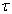, we want to estimate the value of x using the Bayes rule for conditional probability: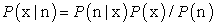(9)

That is by assuming independent Poisson statistics of spike. The final formula reads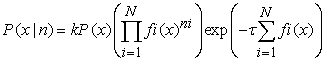(10)

Where k is a normalization constant, P (x) is the prior probability, and fi (x) is the measured tuning function, i.e. the average firing rate of neuron i for each variablevalue x. The most probable value of x can thus be obtained by finding the x that maximizes P (x|n), namely,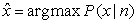(11)

By sliding the time window forward, the entire time course of x can be reconstructed from the time varying-activity of the neural population. The above equation for solving reconstruction problem (corresponding to the most probable value of x) seems to be very similar to the equation searching for optimum solution considering TSP reached by ACS (for random variable S) as follows.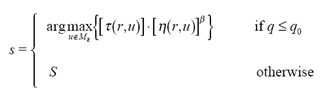(12)

where τ(r,u) is the amount of pheromone trail on edge (r,u), η(r,u) is a heuristic function, which was chosen to be the inverse of the distance between cities r and u, β is a parameter which weighs the relative importance of pheromone trail and of closeness, q is value chosen randomly with uniform probability in [0, 1], q0 (0 ≤q0≤1) is a parameter, Mk is memory storage for k ants activities, and S is a random variable selected according to some probability distribution [1, 4].

### 6. Conceptual Analogy for Three Learning Algorithms Versus ACS Optimization

This section introduces conceptual analogous features considering three deferent adaptive learning algorithms. Namely, they are parallel genetic algorithm, least mean square (LMS) error algorithm, and modified Hebbian algorithm (Oja's rule) applied to search for principal component analysis (PCA). These three learning algorithms are briefly introduced at following sub-sections 6.1, 6.2 and 6.3, respectively.

6.1. Parallel Genetic Algorithm 

The following figure shows the relations between increasing of generations' number versus the misclassification error. It is observed that the given graph behaves similarly as ant colony optimization solution for TSP at Figure 7, given in the above.Download asVeiw figureFigures index
Figure 8. Illustrates decay curve for misclassification error value versus the increase of generation in parallel genetic algorithm (for some constant population size) adapted from 
6.2. Least Mean Square LMS Algorithm 

The following figure presents the learning convergence process for least mean square error as used for training of ANN models . It is clear that this process performed similarly as ACS searching for minimum tour when solving TSP. Additionally, it obeys the performance observed during psycho experimental work carried for animal learning .Download asVeiw figureFigures index
Figure 9. Idealized learning curve of the LMS algorithm adapted from 
6.3. Oja's Rule Algorithm Searching For PCA [15,25]

Referring to the statistical nature of learning processes, , a dynamic recognition system is presented. This system is based on the principal component analysis (PCA) or Karhunen-Loeve transform which is a mathematical way of determining that linear transformation of a sample of points in N-dimensional space which exhibits the properties of the sample most clearly along the coordinate axes. Along the new axes the sample variances are extremes (maxima and minima), and uncorrelated. The name comes from the principal axes of an ellipsoid (e.g. the ellipsoid of inertia), which are just the coordinate axes in question. Additionally that system continuously enlarges in real time, and it is possible to recompute PCA using an iterative gradient search method . This iterative steps (computing eigen values λi) corresponds to increasing of eigen vectors (ei) rank, derived from some randomized data set.

The following figure illustrates the conversion of searching process to obtain PCA for a given randomized data set vectors. At this figure it is noticed that, the magnitude of λi equals the variance in the data set that is spanned by its corresponding ei. So, it is obvious that higher order eigenvectors account for less energy in the approximation of data set since their eigen value have low magnitude corresponding to better signal to noise ratio (S/N).Additionally. shown figure in below agrees with learning performance of other dynamical biophysical model for synaptic plasticity that simulates conditional principal component analysis CPCA, .Download asVeiw figureFigures index
Figure 10. Obtained ordered eigenvalues of data set randomized vectors adapted from 

### 7. Conclusions and Discussions

However, the presented work is mainly concerned with non-human creatures, modeling of human learning performance and memorization has been considered at some recently published research work [8, 28]. Additionally, comparative analogy of quantified learning creativity in humans versus behavioral learning performance of some animals is suggested at . The main conclusion of this presented work is that behavioral intelligence & learning phenomena is based on biological information processing and originated by adaptive (unsupervised) behavioral response during learning by interaction with environment . The mathematical interpretation of that learning phenomenon is given by modified Hebbian learning rule as shown at the above section 2 and Figure 1. That is by autonomous training of random data set in accordance with the statistical nature of behavioral learning processes. All of above leaning algorithms seem commonly closely related (at different levels) to solving of pattern recognition problem. Moreover, the noticed decay of eigenvalues is analogous to decrease of error (learning convergence) at some learning models. That case is analogous to time response (for some other models) and minimum optimum distance for model. Additionally Cramer Rao bound and minimum values of LSM algorithm are analogous to each other. Also, the increase of CPU time is analogous to the increase of stored experience [2, 8]. That means by number of trails at Pavlov and Thorndike work, the store experience correspondence to increase of number of neurons at rat's hippocampus brain area considering pulsed neural system. Finally the obtained result are encouraging for reaches to build up realistic practical models. Finally, considering above learning paradigms and the adopted introduced conceptual view, this opens future research (Integrating Science & Technology) to simulate systematic investigations for biological observations. That is concerned with behavioral learning phenomena for similar creatures' communities including all types of non-human and human creatures as well. [3-8,12,20,28,29,30]..

### References

  H. M. Hassan. “On principles of biological information processing concerned with learning convergence mechanism in neural and non-neural bio-systems”, Published at CIMCA 2005 28-30 Nov. 2005.In article View Article  Dorigo, M., & Gambardella, L. M. (1997). Ant colony system: a cooperative learning approach to the travelling salesman problem. IEEE Transactions on Evolutionary Computation, 1(1), 53-66.In article View Article  Dorigo, M.: Optimization learning and natural algorithms, (in Italian), Ph.D Thesis Dip. Electronico, Politecnico di Milano, (1992).In article  Dorigo, C, M., Maniezzo, V. : Distributed optimization by Ant Colonies. In: Proceedings of The First European conference on Artificial Life (ECAL 91), pp 134-142 Elevier, (1991).In article  Dorigo, M., Maniezzo, V. : Positive feedback as search strategy. Technical report 91-016, Dip .Electronico, Politecnicodi Milano, (1991).In article  H. M. Hassan. “On Learning Performance Evaluation for Some Psycho-Learning Experimental Work versus an Optimal Swarm Intelligent System.”, Published at ISSPIT 2005 (18-20 Dec.2005).In article  H. M. Hassan “Comparative Performance Analysis for Selected Behavioral Learning Systems versus Ant Colony System Performance (Neural Network Approach)”. Published at the International Conference on Machine Intelligence (ICMI) 2015.Held on Jan 26-27, 2015, in Jeddah, Saudi Arabia.In article  Hassan M. H. Mustafa, et al. “Comparative Performance Analysis and Evaluation for One Selected Behavioral Learning System versus an Ant Colony Optimization System” Published at the Proceedings of the Second International Conference on Electrical, Electronics, Computer Engineering and their Applications (EECEA2015), Manila, Philippines, on Feb. 12-14, 2015.In article  Thorndike E.L. Animal Intelligence, Darien, Ct. Hafner, 1911.In article PubMed  Pavlov, I.P. Conditional Reflex, An Investigation of The Psychological Activity of the Cerebral Cortex, New York , Oxford University press, 1927.In article  Hampson, S.E. Connectionistic Problem Solving, Computational Aspects of Biological Learning, Berlin, Birkhouser, 1990.In article View Article  H.M. Hassan. “A Comparative Analogy of Quantified Learning Creativity in Humans Versus Behavioral Learning Performance in Animals: Cats, Dogs, Ants, and Rats. (A Conceptual Overview), submitted at WSSEC08 conference to be held on 18-22 August 2008, Derry, Northern Ireland.In article  Fukaya, M., et al. Two level Neural Networks: Learning by Interaction with Environment, 1st ICNN, San Diego, 1988.In article  Ghoaimy M. A., et al. learning of Neural Networks using Noisy Data, Second International Conference On Artificial Intelligence Application, Cairo, Egypt, 389-399, Jan. 22-24, 1994.In article  David J., Jilk, Danial M. Cer, and Randall C. O'Rilly., “Effectiveness of neural network learning rules generated by a biophysical model of synaptic plasticity.”, Technical report, Department of Psychology, University of Colorado, Boulder, (2003).In article  Mariaty, E.D et al, 1999: Evolutionary algorithms for reinforcement learning, journal of AI research 11:241.In article  Folino G., Pizzuti C. and Spezzano G. Parallel genetic programming for decision tree induction. Technical Report ISI-CNR, Università della Calabria, 87036 Rende (CS), Italy, 2002.In article  Sejnowski, T.J: Neural pulse coding” foreword article for (Pulsed neural networks), MIT press, 1999, pp 13-23, 1999.In article  Wilson, M. A. and McNaughton, B. L., 1993: Dynamics of the hippocampal ensemble code for space. Science, 261: 1055-8.In article View Article  PubMed  H. M. Hassan. “Evaluation of Learning / Training Convergence Time Using Neural Network (ANNs)” published at, the Proceeding of 4th International Conference of Electrical Engineering ICEENG Conference, Military Technical College, Cairo, Egypt, pp.542-549, 24-26 Nov. 2004.In article  H.M. Hassan “On Quantifying Learning Creativity Using Artificial Neural Networks (A Mathematical Programming Approach)” published at CCCT 2007 conference held on July 12-17,2007-Orlando,Florida,USA.In article  Haykin, S. Neural Networks, Englewood Cliffs, NJ: Prentice-Hall pp 50-60, 1999.In article  Zhang et al. “Interpreting neuronal population activity by reconstruction.” Journal of Neurophysiology, 79:1017-44, 1998.In article PubMed  Hebb, D.O. “The organization of Behavior, A Neuropsychological Theory”, New York, Wiley, 1949.In article PubMed  Roseborough and Murase. at web site http://www-white.media.mit.edu/people/jebara/uthesis/node64.html, 2000.In article  Jebara T., 2000: at web site http://www-white. media.mit. edu/people/jebara/ uthesis/node64.html.In article  Alberto C., et al. Distributed optimization by ant colonies. Proceeding of ECAL91, Elsevier Publishing, pp 134-142, 1991.In article  H.M. Hassan, Ayoub Al-Hammadi, Bernd Michael” Evaluation of Memorization Brain Function Using a Spatio-temporal Artificial Neural Network (ANN) Model” published at CCCT 2007 conference held on July12-15 ,2007 – Orlando, Florida, USA.In article